# III. 2MASS Details

## 3. Data Processing

### e. Position Reconstruction

#### Position Reconstruction Technique

Table 3: Formulation of Least-Squares Problem

The total sum "S" of weighted differences squared can be broken down into three components:

(a) frame-to-frame differences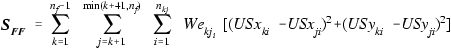(Eq. III.3.e.1)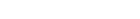where

nf = number of frames in scan
nkj = number of points common to frames k and j
Wekji = inverse variance weighting for ith common point between frames k and j
USxki = U-scan x coordinate of frame k source associated with point i of the kj set of common points
USxji = U-scan x coordinate of frame j source associated with point i of the kj set of common points
USyki = U-scan y coordinate of frame k source associated with point i of the kj set of common points
USyji = U-scan y coordinate of frame j source associated with point i of the kj set of common points;

(b) frame-to-reference differences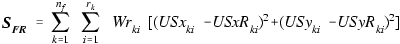(Eq. III.3.e.2)where

nf = number of frames in scan
rk = number of reference stars matched to frame k
Wrki = inverse variance weighting for ith reference match to a frame k source
USxki = U-scan x coordinate of frame k source associated with ith reference match within frame
USyki = U-scan y coordinate of frame k source associated with ith reference match within frame
USxRki = U-scan x coordinate of ith reference star matched within frame k
USyRki = U-scan y coordinate of ith reference star matched within frame k;

and,

(c) relative a priori frame-to-frame differences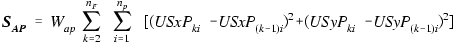(Eq. III.3.e.3)where

nf = number of frames in scan (only J2 frames used)
np = number of artificial pegs in each frame-to-frame overlap
Wap = arbitrarily small weighting factor
USxPki = U-scan x coordinate of peg from frame k associated with ith common point of the (k-1, k) set
USyPki = U-scan y coordinate of peg from frame k associated with ith common point of the (k-1, k) set
USxP(k-1)i = U-scan x coordinate of peg from frame k-1 associated with ith common point of the (k-1, k) set
USyP(k-1)i = U-scan y coordinate of peg from frame k-1 associated with ith common point of the (k-1, k) set.

The third set of differences is weighted very lightly and is intended to bridge gaps. Three artificial stars or "pegs" are added between each J2 frame and the next. The "pegs" are placed in a triangular pattern within the a priori frame-to-frame overlaps, with one set between J2 frames 1 and 2, a second set between frames 2 and 3 and so on. With all frames in their a priori positions, these differences are all zero.

In order to formulate the required set of these simultaneous equations,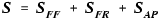(Eq. III.3.e.4)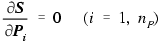(Eq. III.3.e.5)where

SFF = frame-to-frame sums
SFR = frame-to-reference sums
SAP = a priori J2-frame-to-J2-frame sums
Pi = parameter i
nP = total number of parameters,

partial derivatives of S with respect to each of the 563 adjustment parameters are set to zero.